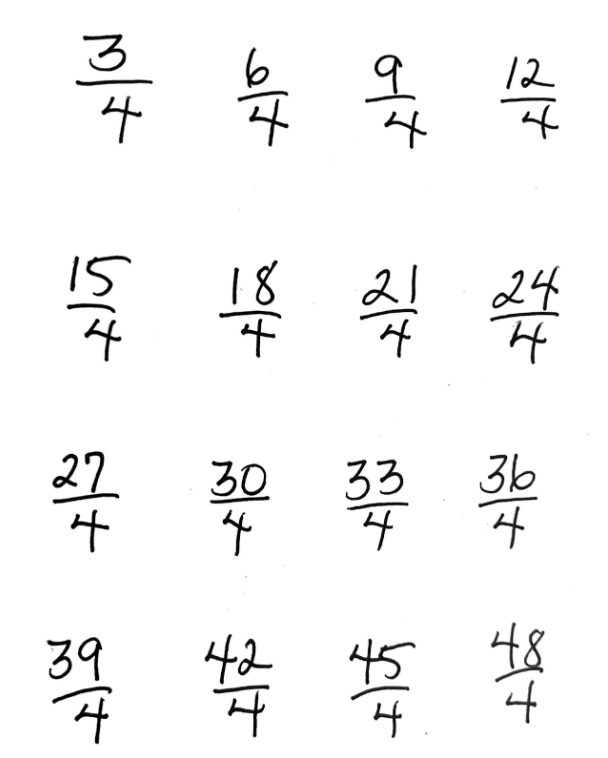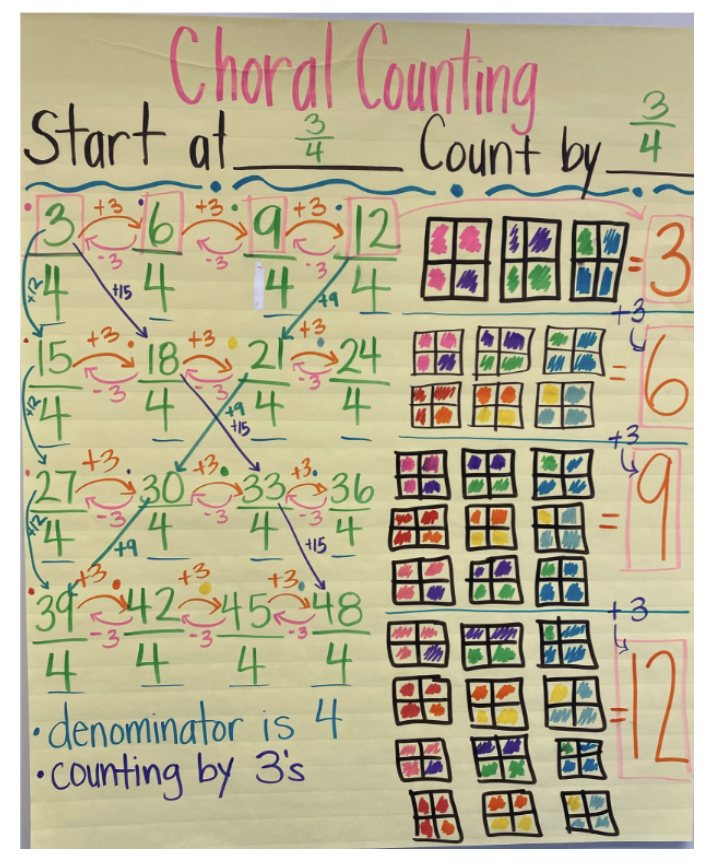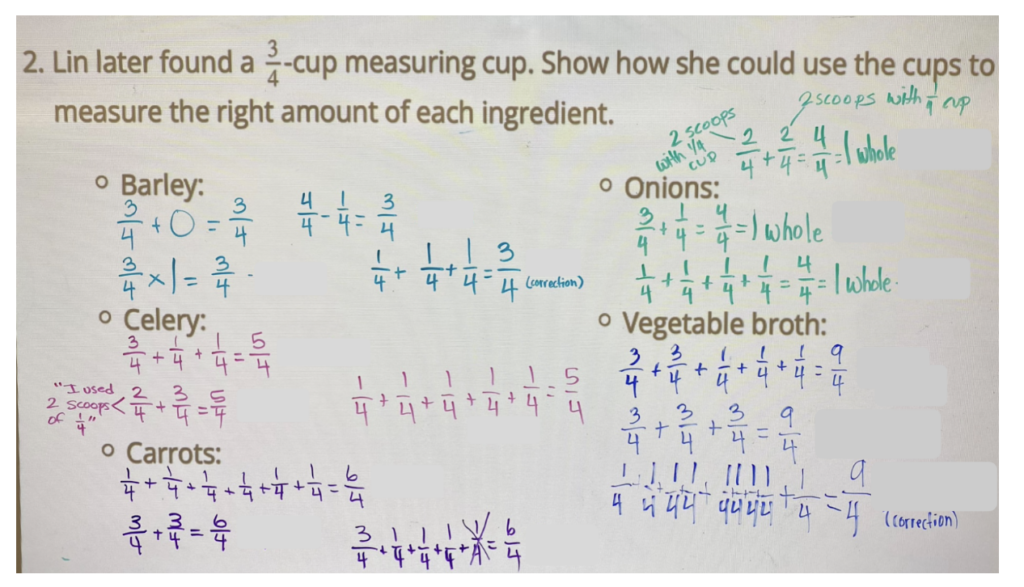# Enacting IM K–5 Math™ Lessons in a Grade 4 and 5 Special Education Class

By Jody Guarino, Math Coordinator and Suzanne Huerta, SDC Teacher

## What does it look like for everyone in a school to learn together?

This question, posed by Elham Kazemi, pushes us to consider that we, adults and educators, can learn collaboratively with the students in our classrooms. We situate this blog in our experience as educators learning with and from students. This represents the collaboration of (1) Suzanne, a special education teacher in Buena Park School District; (2) the students in her self-contained grade 4 and 5 special education class; and (3) Jody, a math teacher educator.

Fractions make up a significant part of upper elementary math content. This content is an important foundation for future math and strongly linked to success with algebra, even more so than socioeconomic status, IQ, and family education (Siegler et al, 2012). Knowing this, it’s important to us to consider how we can make sure all students have access, especially students with disabilities.

Suzanne’s fourth and fifth grade students were working on operating with fractions. Let’s examine one lesson in Suzanne’s class. How did she modify the lesson to ensure all students have access? And what did everyone—teacher, students, and math teacher educator—learn through this collaboration?

### Enacting the Warm-Up

The lesson (Grade 4 Unit 3 Lesson 7) begins with the choral counting routine. Students count by $\frac{3}{4}$ beginning at  $\frac{3}{4}$ and counting until reaching $\frac{48}{4}$. Numbers would be written 4 to a row.How could we support students in making sense of these numbers? Suzanne planned to stop at the end of each row and invite students to visually represent the last fraction. Visual representations support students to develop a deeper understanding of the fractions they are working on as they process the information.As Suzanne and her students counted chorally, she charted each number. When she reached $\frac{12}{4}$ (see choral counting chart) she asked, “How can we represent $\frac{12}{4}$? Watson responded, “draw two boxes and partition them into fourths.” Kerry added on, suggesting color coding each fraction with a dot next to it and then color in 3 parts with that color.

Within this interaction, we noticed the precise language Watson used to partition: fourths. We also noticed the affordance of the suggested color coding to make each $\frac{3}{4}$ visible, supporting students to make meaning of the four $\frac{3}{4}$ within $\frac{12}{4}$. As the suggested representation was being drawn, students were quick to realize the need to draw another box and partition it in fourths in order to represent each fraction and its corresponding color.

The count then continued from $\frac{15}{4}$ with students again stopping at the end of each row to add representations. The visual representations of fractions allowed students with disabilities to make sense of their mathematical thinking and make it more concrete.

### Barley Soup

Next came Activity 1: Barley Soup. At first, the task calls for making the given recipe with only a $\frac{1}{4}$ measuring cup. Before getting started with the activity, Suzanne asked her students what their favorite soup was, what ingredients are in their favorite soup, and what a soup recipe would look like. Students contributed ideas and shared experiences making and eating soup! The context of cooking was familiar to students and offered a point of access. They were hooked from the very start of the lesson!

As a class, they looked over the barley soup recipe and discussed the different ingredients, some they were familiar with, and others that were new to them. Suzanne then pulled out a $\frac{1}{4}$ measuring cup and asked students how they might use the measuring cup to scoop out the ingredients. Students worked in groups of 3, with each group getting a $\frac{1}{4}$ cup. While they didn’t have the actual ingredients, many students used the measuring cup and a scooping motion as they solved the problem.

Rich, meaningful, mathematical conversations occurred. Aiden and Jeremy were discussing how they needed to figure out how to get $\frac{3}{4}$ cups of barley using only a $\frac{1}{4}$ cup. Aiden picked up the $\frac{1}{4}$ cup and “scooped” $\frac{1}{4}$ cups of barley in the air and poured it into an imaginary bowl. He scooped another and said, “$\frac{1}{4}$, $\frac{2}{4}$. Two scoops is $\frac{2}{4}$. If we add one more scoop that will be $\frac{3}{4}$!” He scooped another $\frac{1}{4}$ cup of barley into his imaginary bowl and he and Jeremy cheered with excitement and quickly moved on to figure out how many scoops of a $\frac{1}{4}$ cup will they need for $\frac{5}{4}$ cups of chopped celery. In this exchange, and among other groups of students, their feeling of success was evident. Students had smiles on their faces and the classroom was buzzing. As students worked, they used the $\frac{1}{4}$ measuring cup to “scoop” each ingredient. Students then recorded their thinking, some counting the number of $\frac{1}{4}$’s, others using repeated addition.

Next, each group was given a $\frac{3}{4}$ cup in addition to their $\frac{1}{4}$ cup. Students were asked to measure out the right amount of ingredients using both the $\frac{1}{4}$ cup and the $\frac{3}{4}$ cup. They got right to work, excited to figure out how many scoops each ingredient would take. Again, students shared their thinking with each other as they “scooped” out the ingredients they needed in the air and recorded their equations that matched their strategies. As the class came together, students shared their reasoning and discussed the different ways they wrote expressions as sums of smaller fractions, while Suzanne recorded their thinking (see image below). Students listened intently as ideas were shared and offered ideas that differed from those already shared. They noticed that there were many ways they could scoop out the ingredients, and many ways to compose the quantity called for in the recipe.As we reflect on the lesson, what struck us most was the joy and energy in the classroom. Every student was successful, mathematically and beyond! Students understood the problem, solved in ways that made sense to them, worked collaboratively with peers, and represented their thinking on paper, all as they worked on grade-level math, decomposing and composing fractions from unit fractions and non-unit fractions and representing their compositions with equations. Beyond the math content, students saw themselves and each other as capable knowers and doers of mathematics!

### Conclusion

Throughout our work this spring, we’ve watched students with disabilities enthusiastically engage in grade-level math. We’ve seen what they are able to do when given opportunities. Students are making sense of fractions and fraction operations conceptually. The use of visual representations, familiar contexts, physical tools (such as measuring cups), and opportunities to work collaboratively with peers have led students to make sense of math content that can be challenging for students. While these strategies are often part of the experience for general education students, they are just as important for students with disabilities.

Throughout our collaboration we’ve learned the importance of coherence within and across lessons, and specifically how that coherence provides students with access. In the context of this lesson, the choral count engaged students in working with fourths, attending to representations, combining and counting quantities, and seeing patterns within the number system, all important to the Barley Soup lesson activity. The invitation to the math not only draws students in, it sets them up for success within the lesson. As we see the smiles on students’ faces, and hear the joy in the classroom as they engage in math, we affirmed our belief that all students, including those with disabilities, can do grade-level math, and it is our role as the educators who serve them to provide them with access.